## A political candidate has asked his/her assistant to conduct a poll to determine the percentage of people in the community that supports him

Question

A political candidate has asked his/her assistant to conduct a poll to determine the percentage of people in the community that supports him/her. If the candidate wants a 3% margin of error at a 90% confidence level, what size of sample is needed

in progress 0
2 months 2021-07-23T03:58:39+00:00 1 Answers 1 views 0

A sample size of 752 is needed.

Step-by-step explanation:

In a sample with a number n of people surveyed with a probability of a success of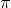, and a confidence level of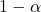, we have the following confidence interval of proportions.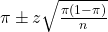In which

z is the z-score that has a p-value of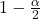.

The margin of error is of: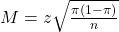90% confidence level

So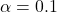, z is the value of Z that has a p-value of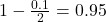, so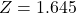.

If the candidate wants a 3% margin of error at a 90% confidence level, what size of sample is needed?

We have no estimate of the proportion, so we use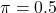.

The sample size is n for which M = 0.03. So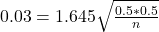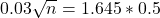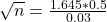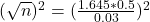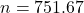Rounding up:

A sample size of 752 is needed.